# Kerala SSLC Board Question Papers for Class 10th Maths 2020 With Solutions In PDF

Kerala SSLC (Class 10) maths 2020 question paper with solutions are provided here in a downloadable pdf format and also in the text so that the students can easily access them. Along with the solutions, they can also get the maths question paper 2020 class 10 SSLC for reference. Students are able to access all the Kerala board previous year maths question papers here. SSLC question paper 2020 Kerala for English medium with answers can be downloaded easily and students can practice and verify the answers provided by BYJU’S. Solving 2020 Maths question papers for class 10 will help the students to predict what type of questions will appear in the exam.

### QUESTION PAPER CODE S 1531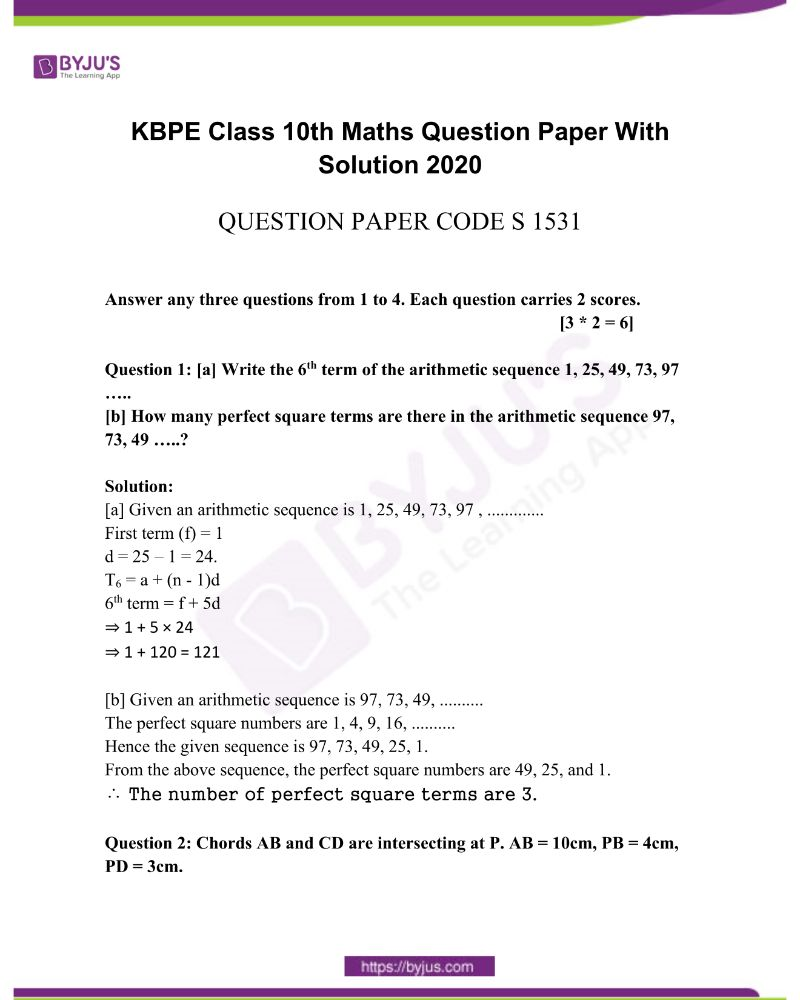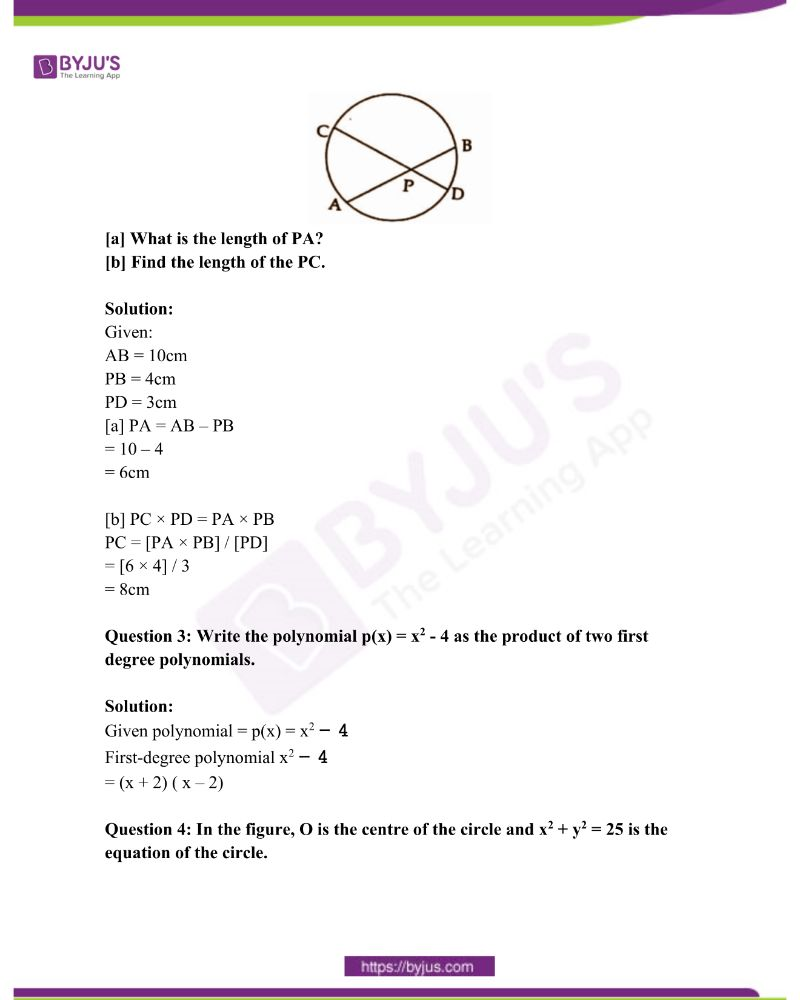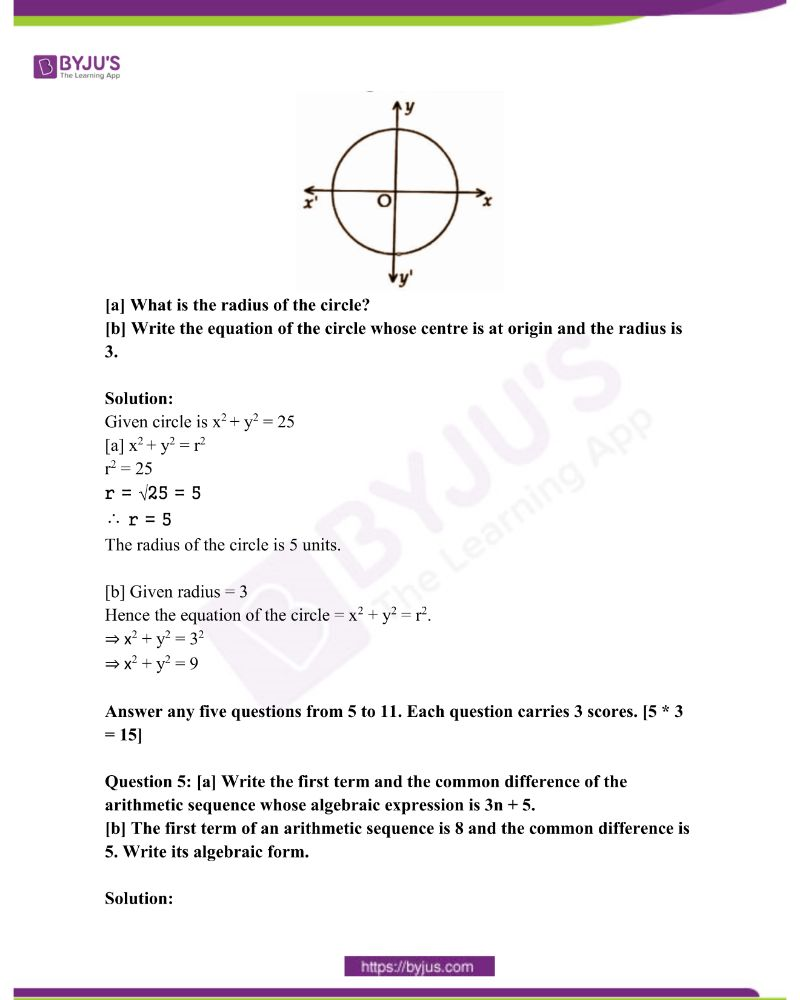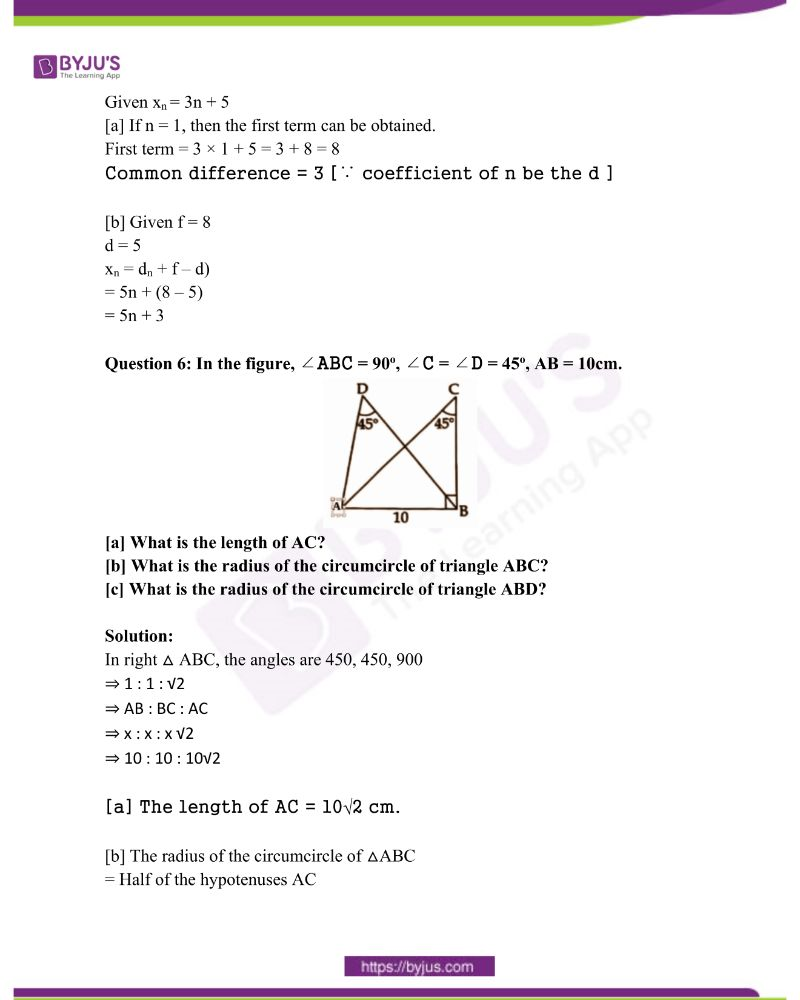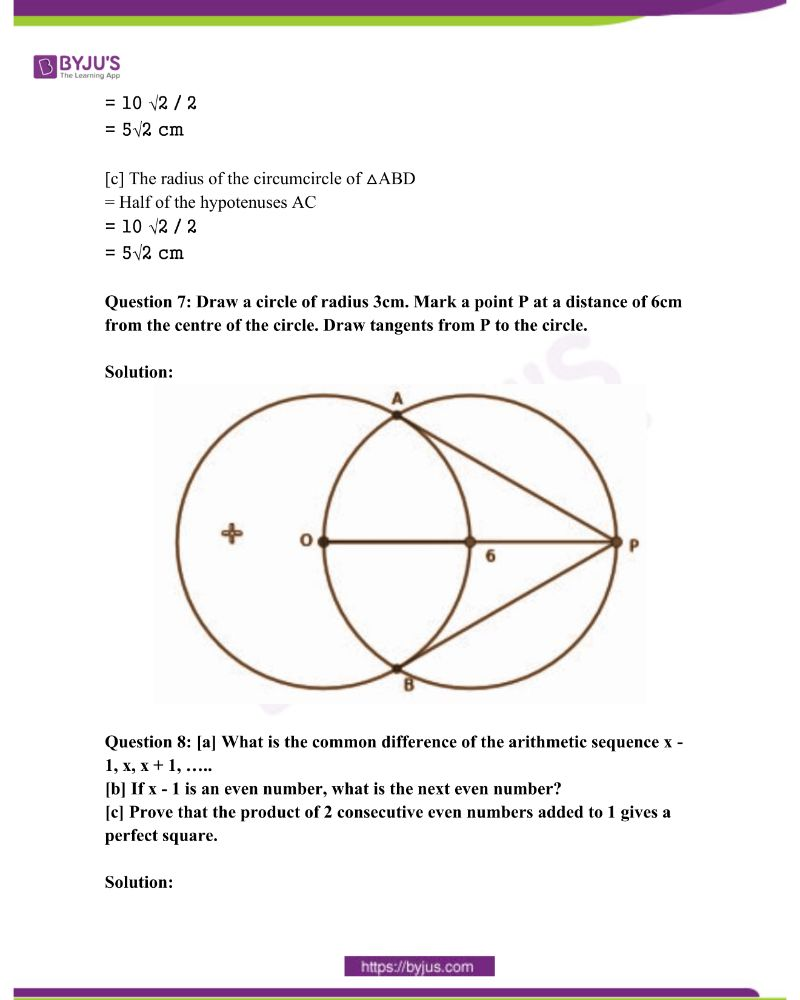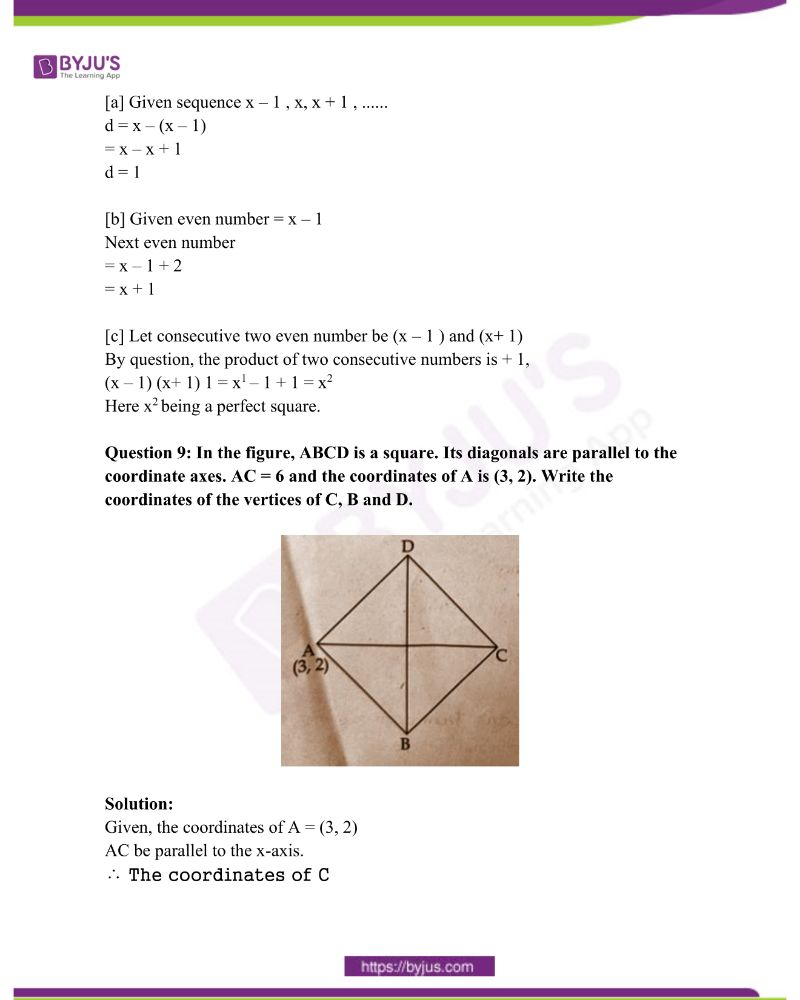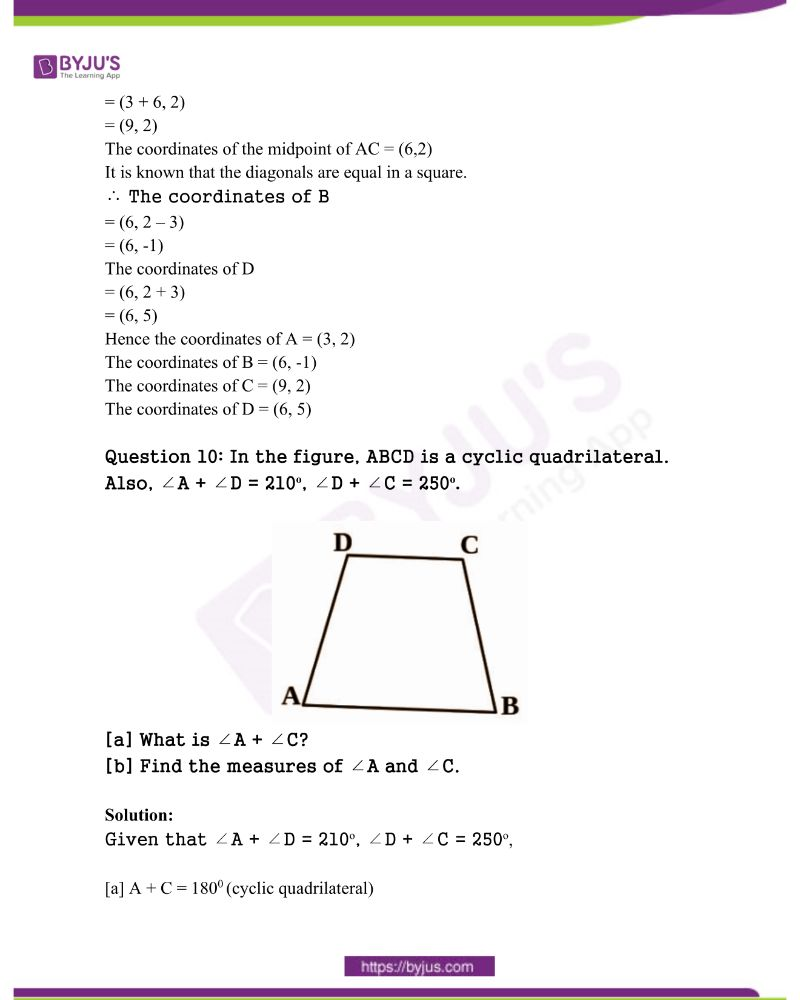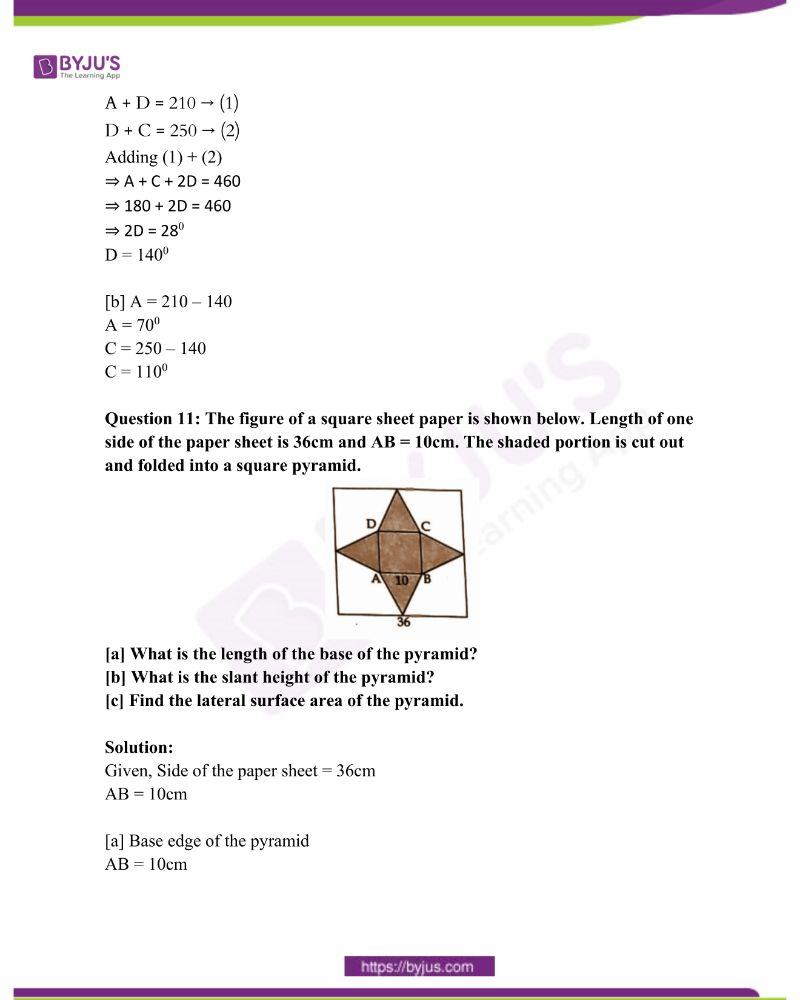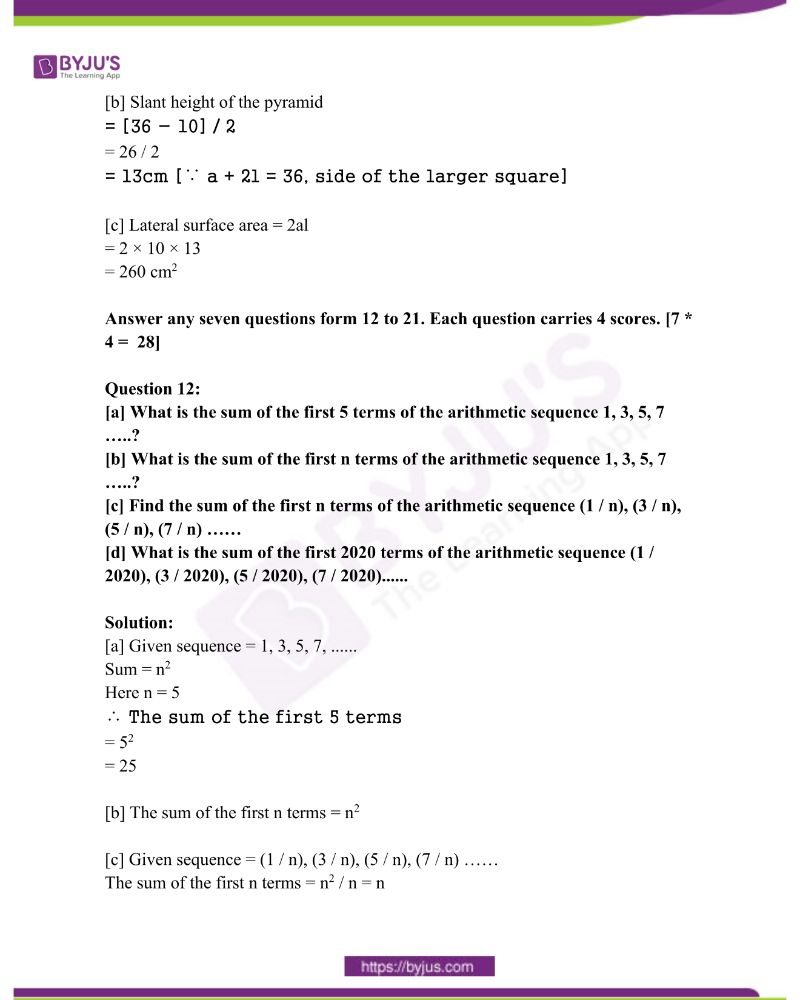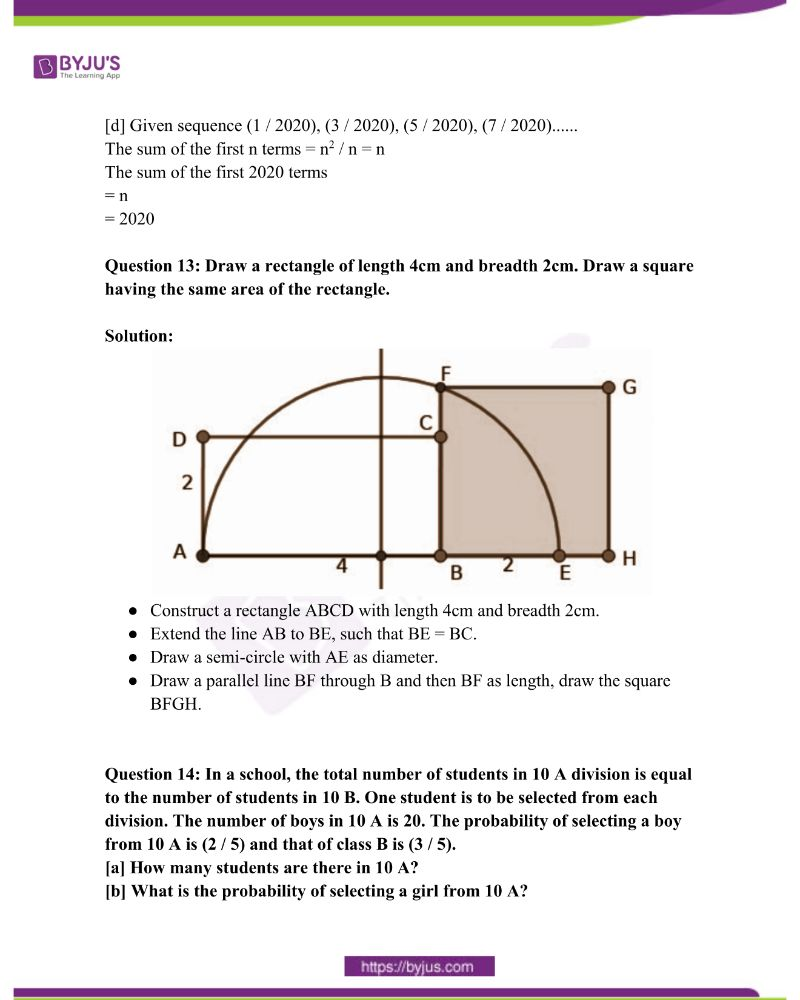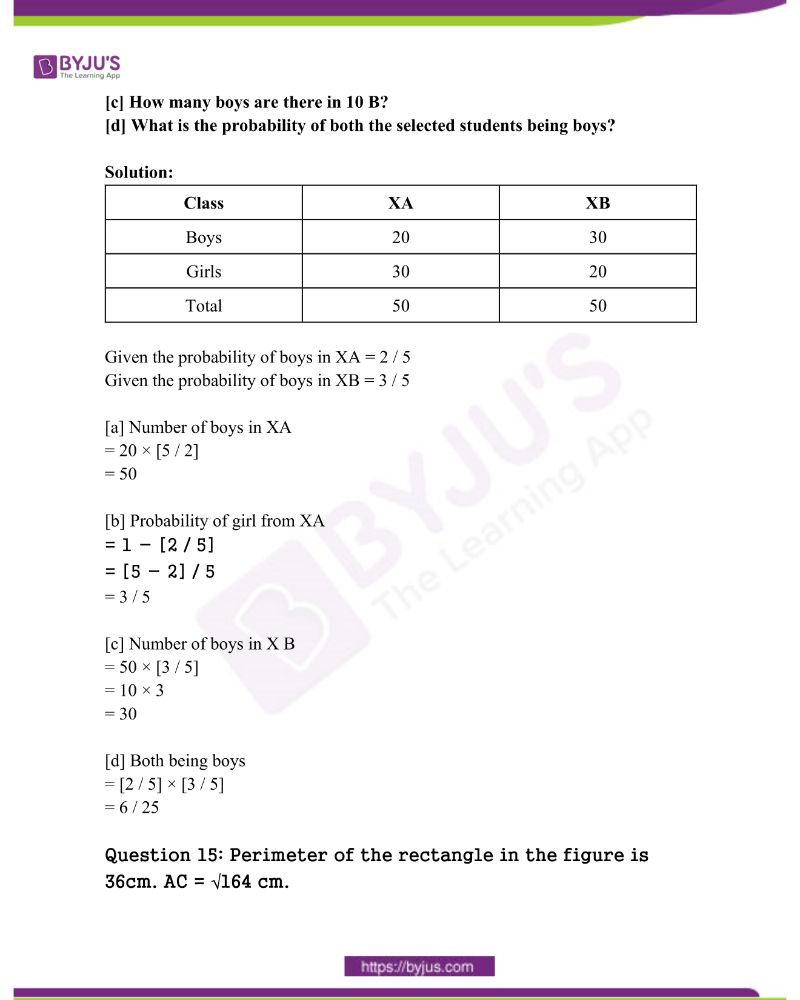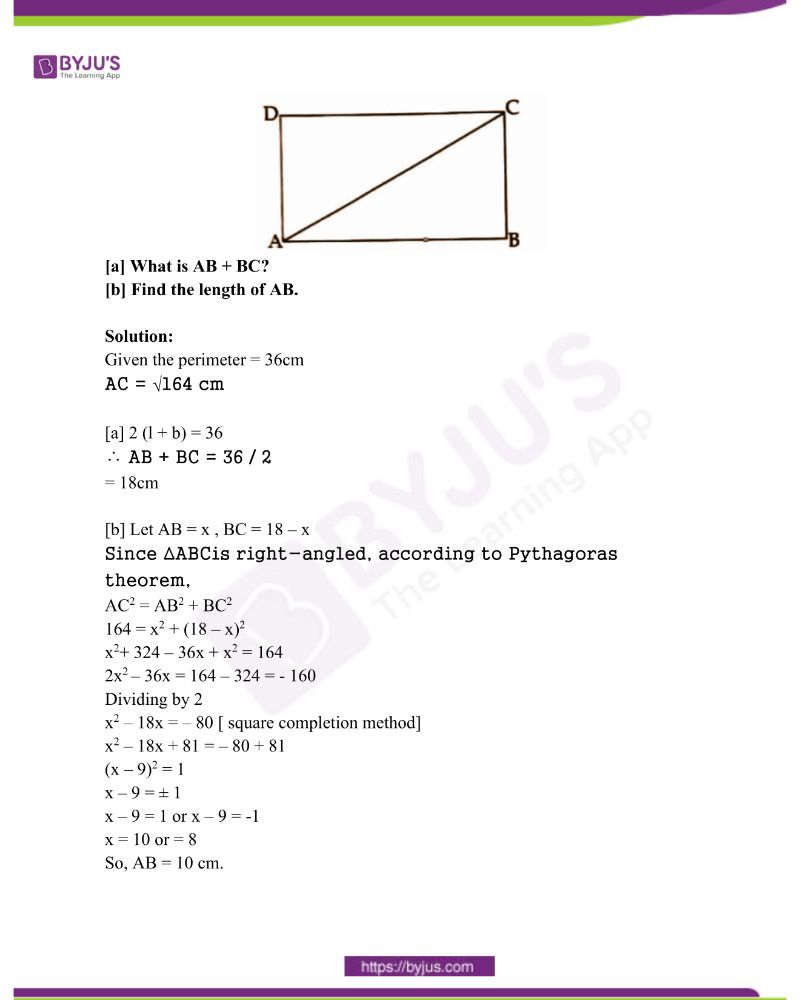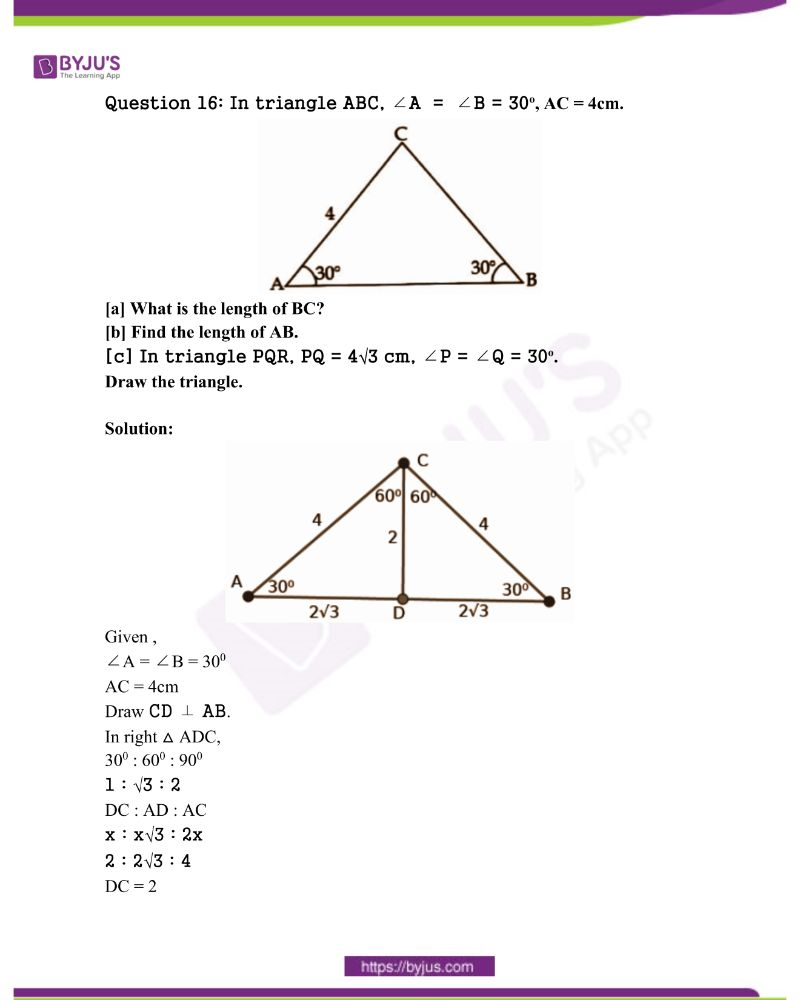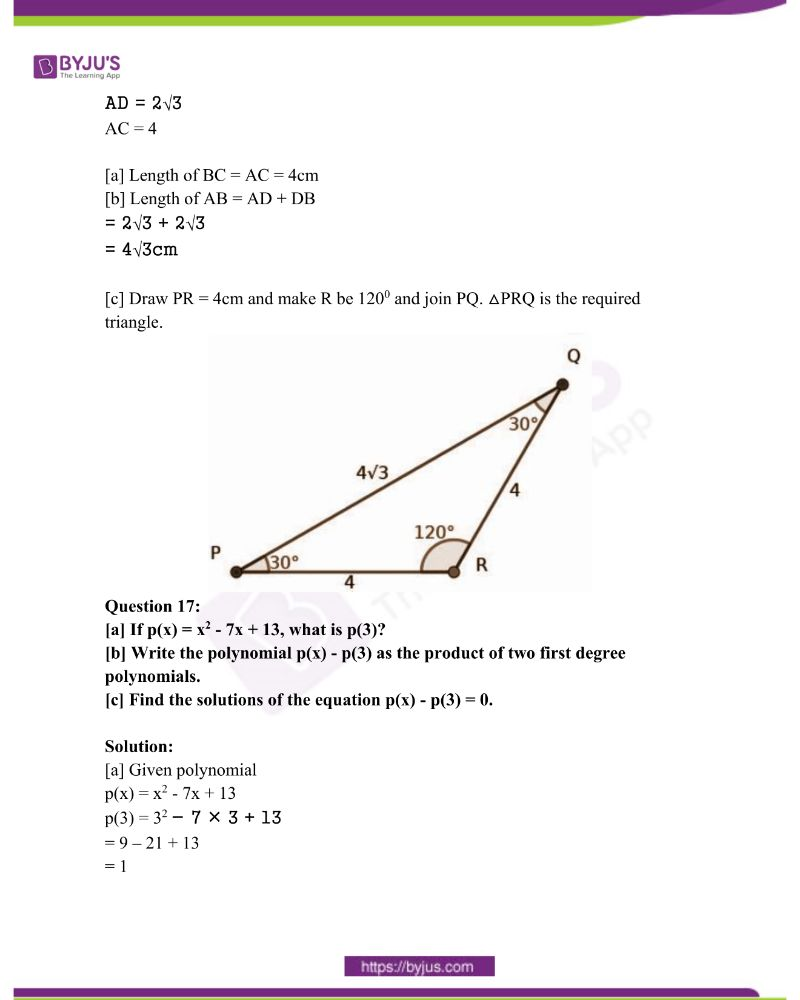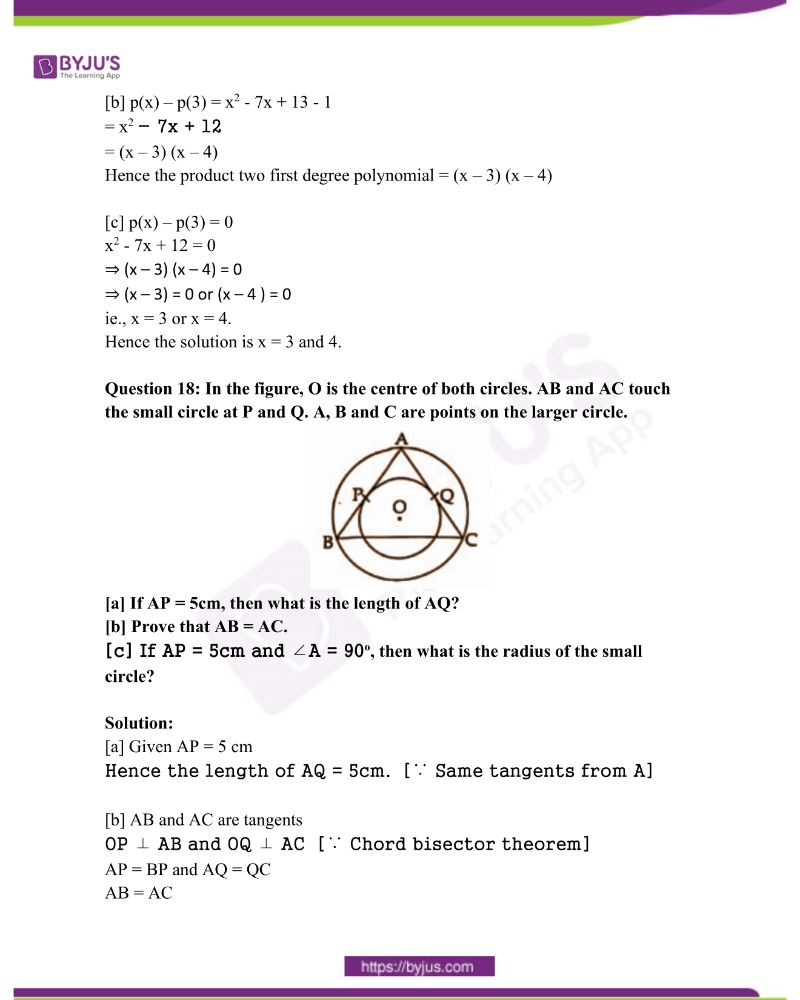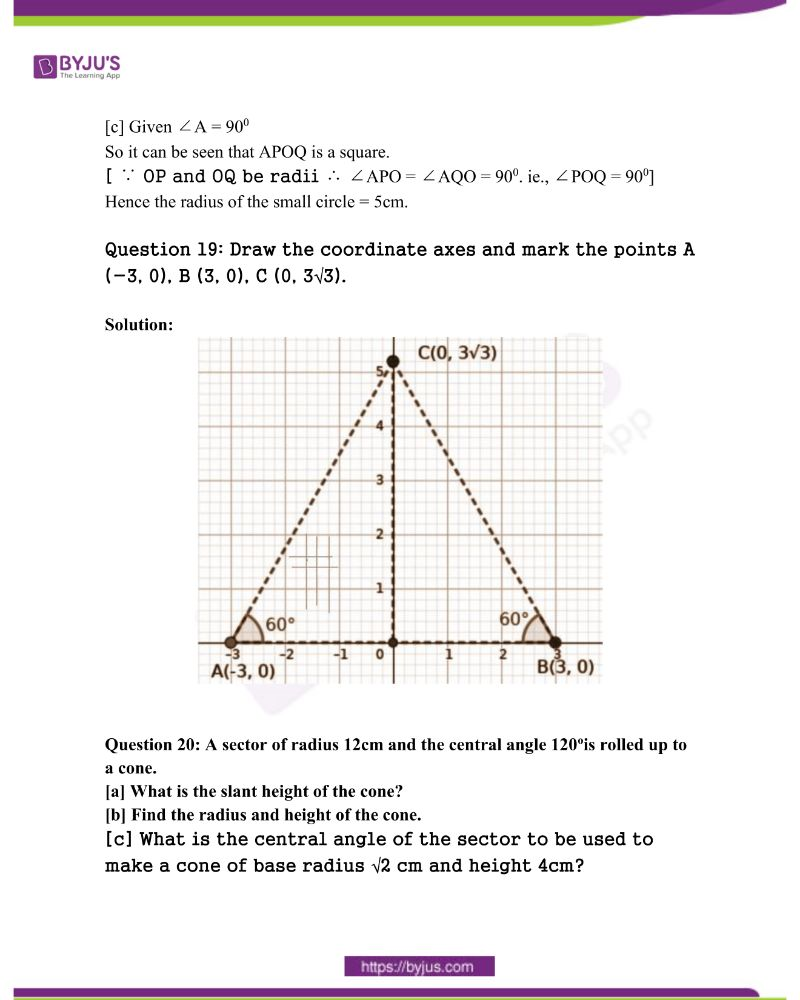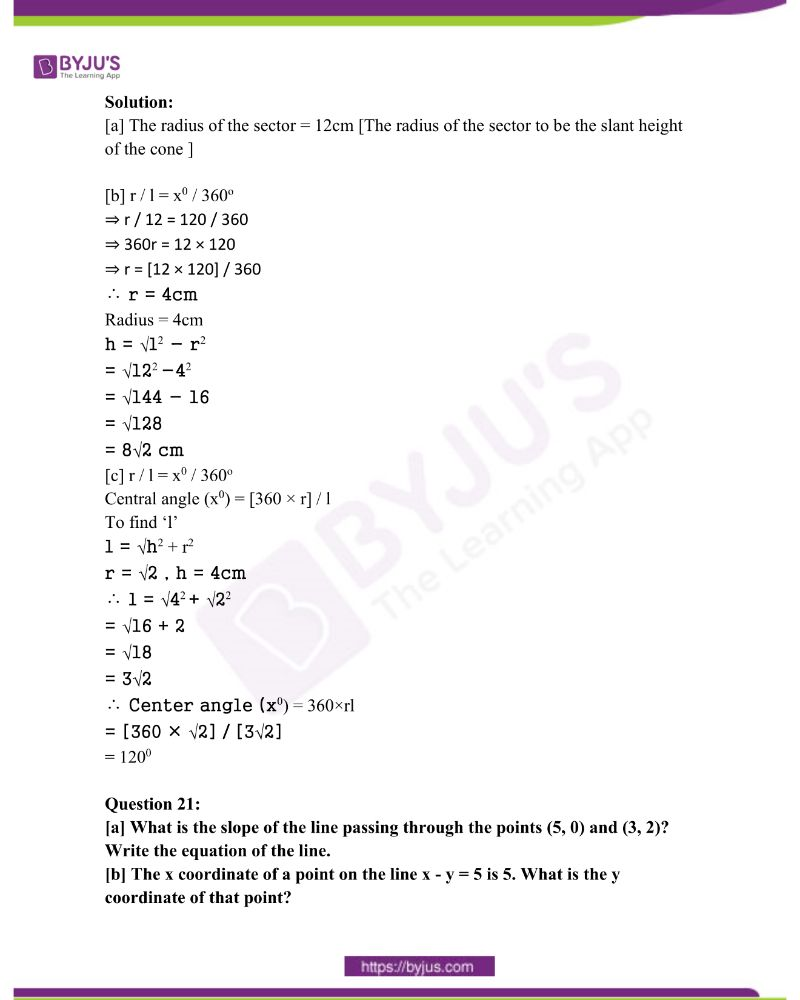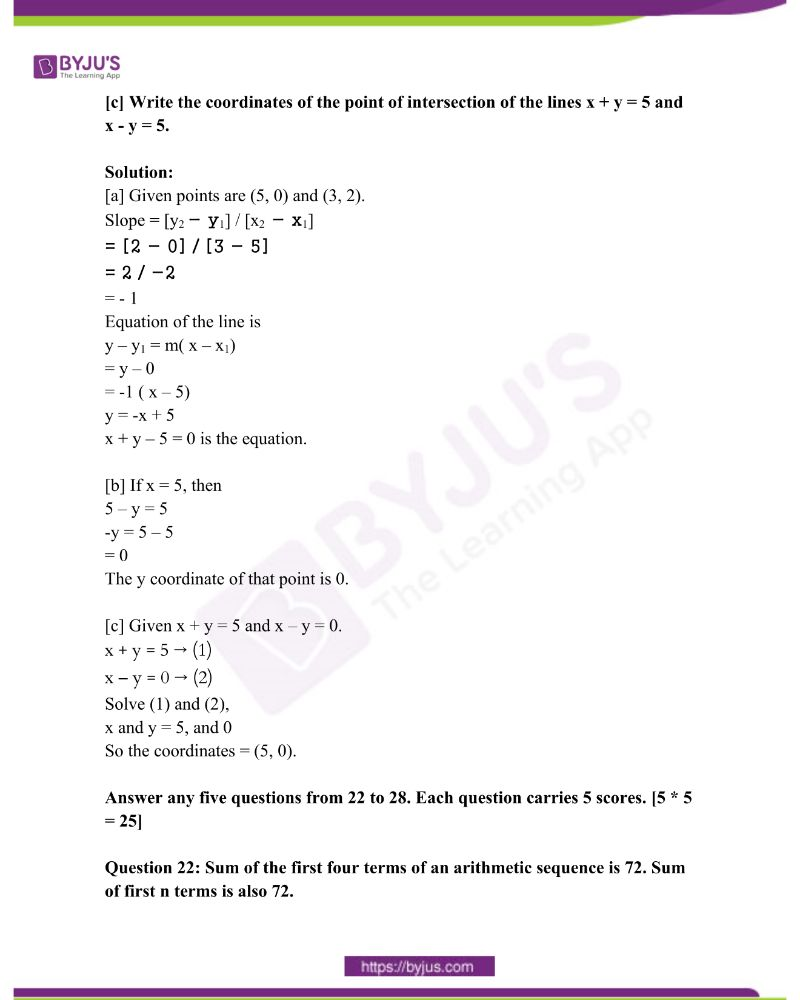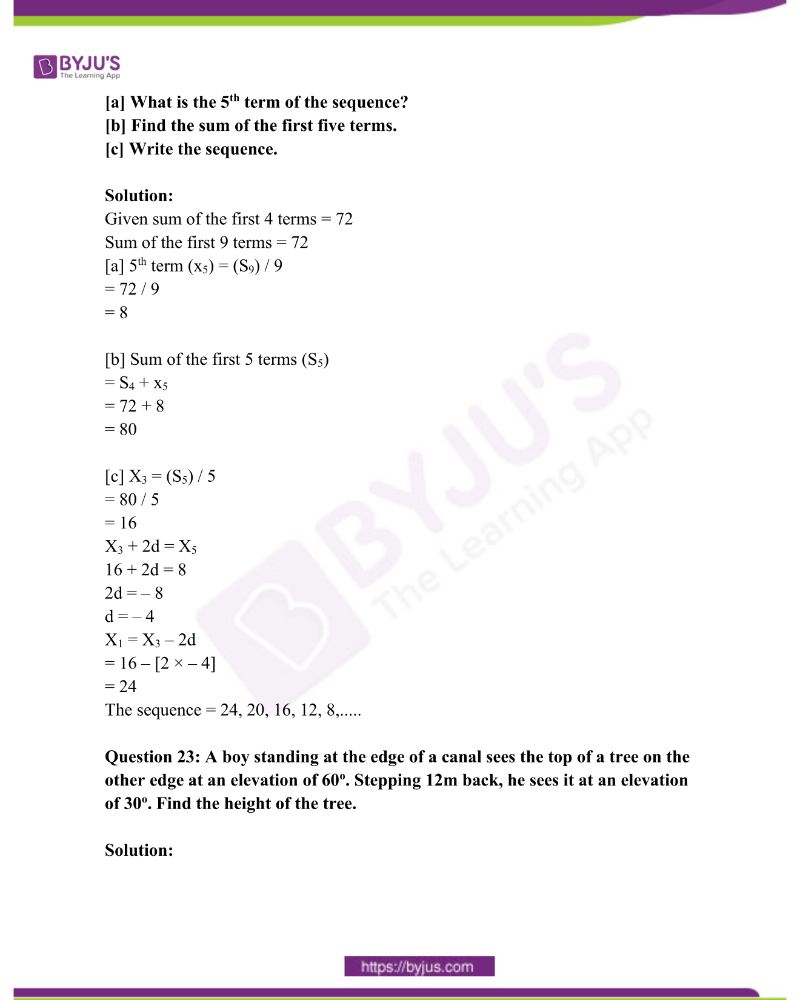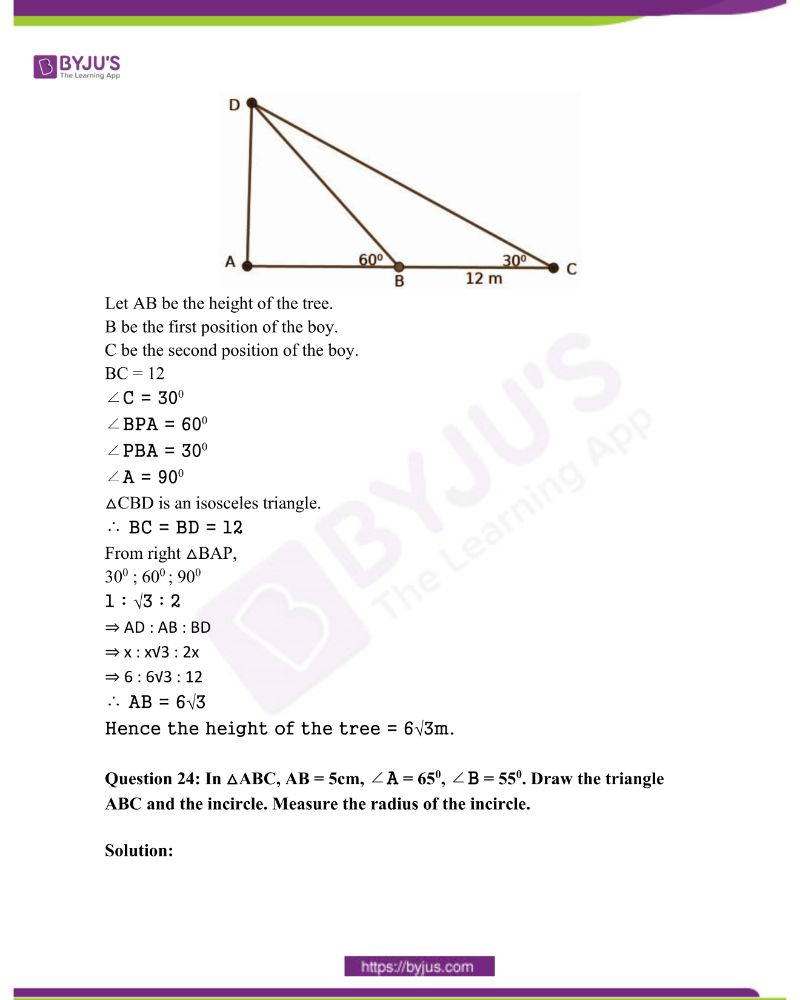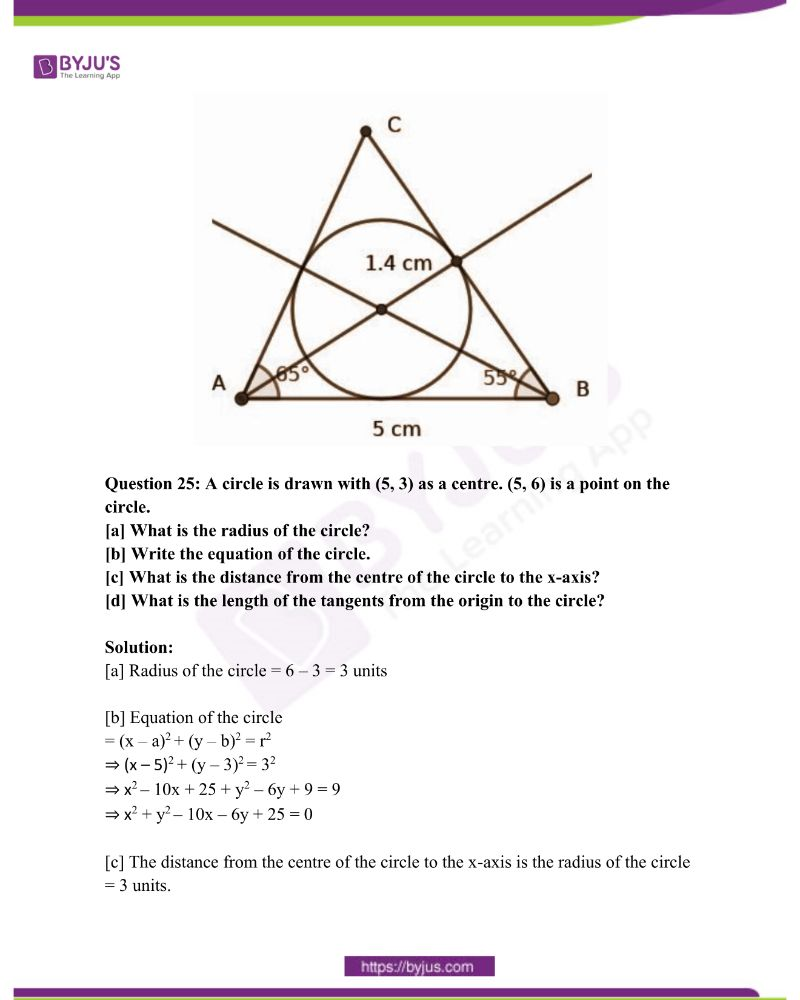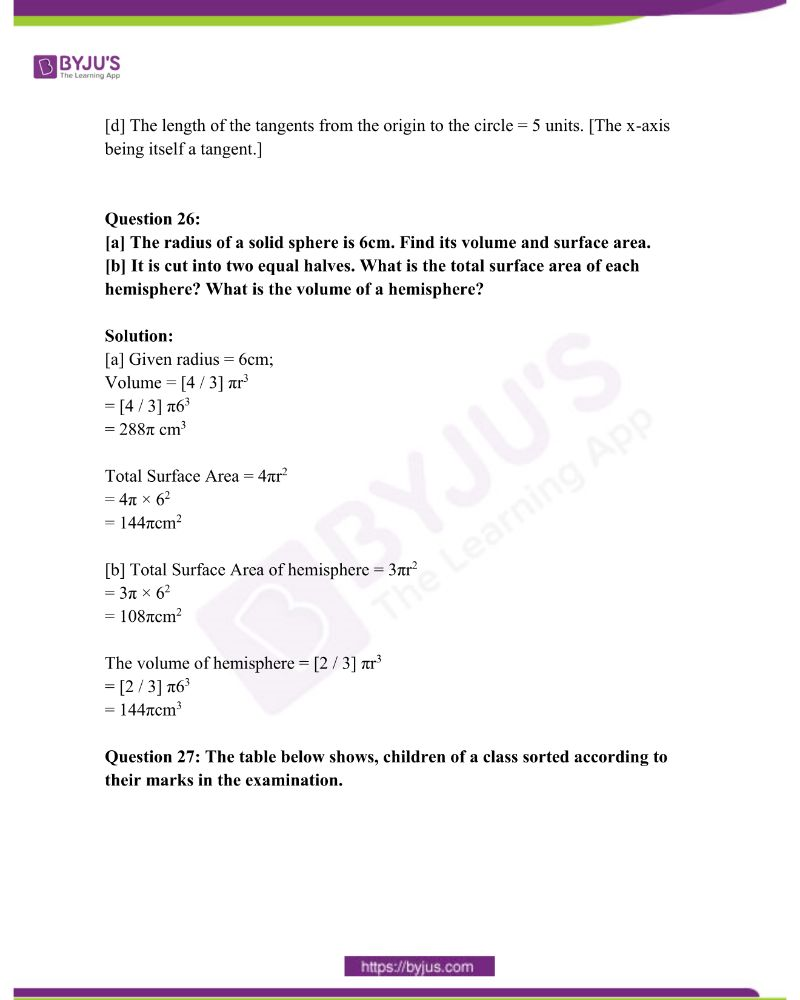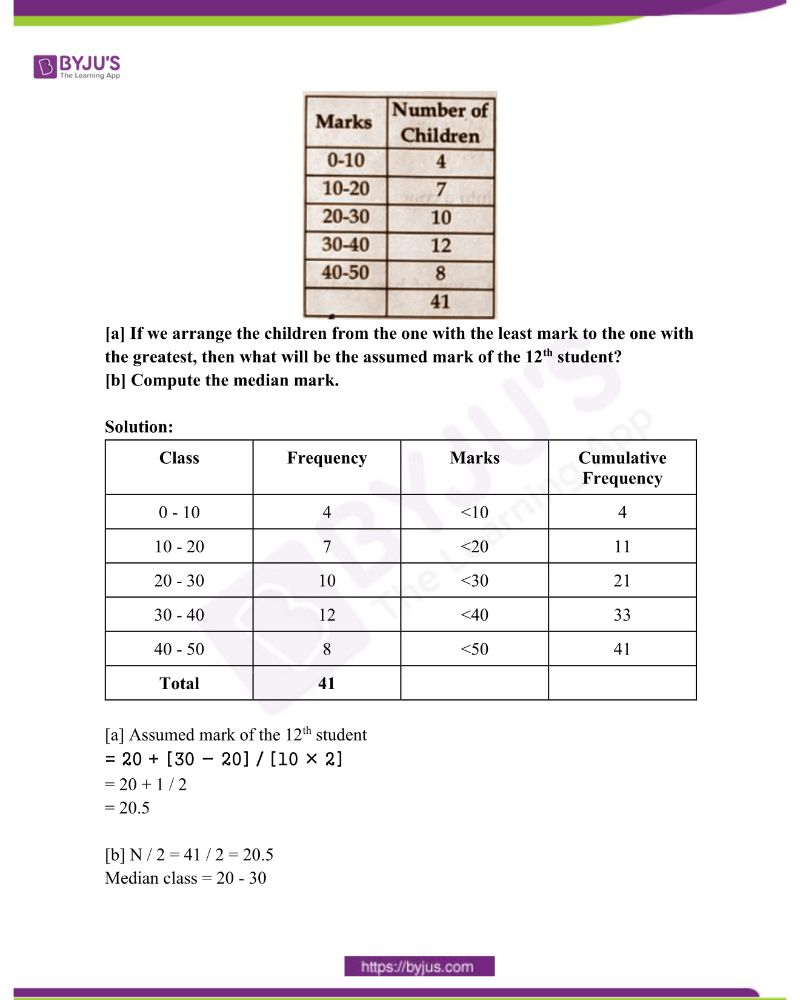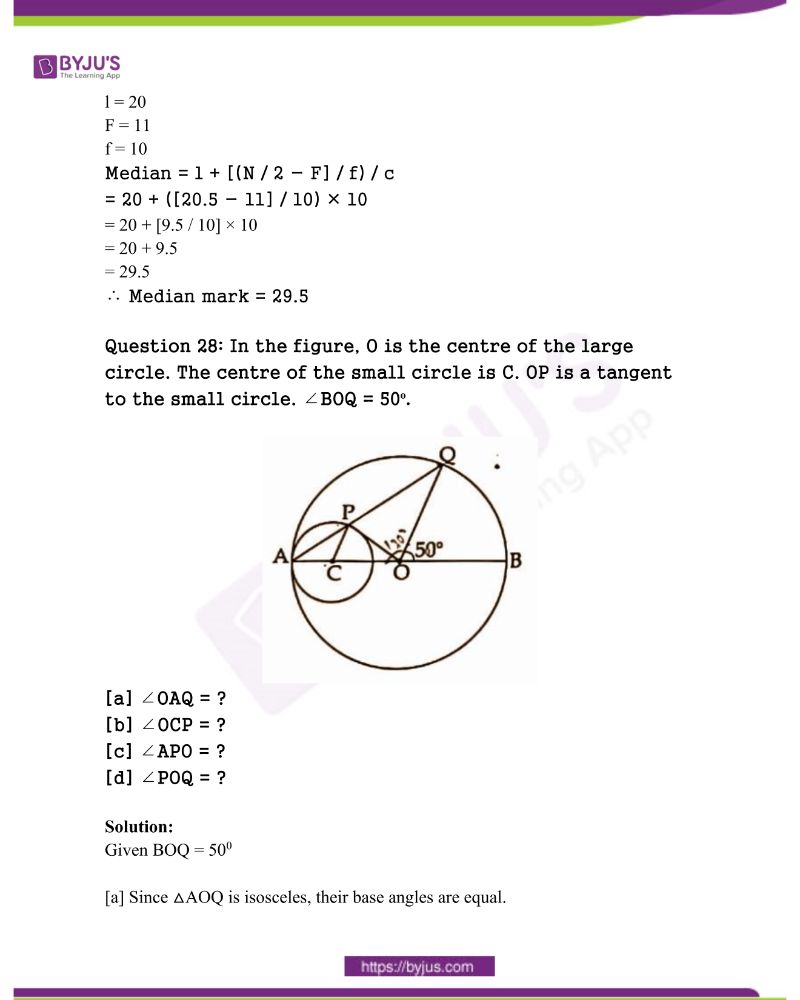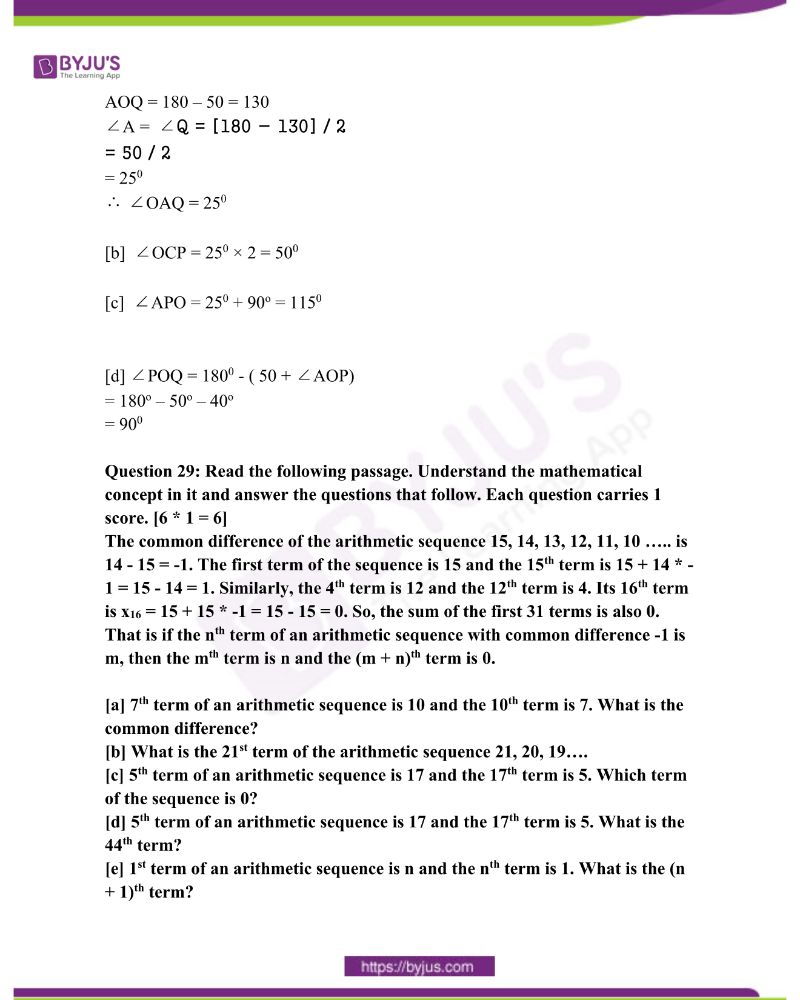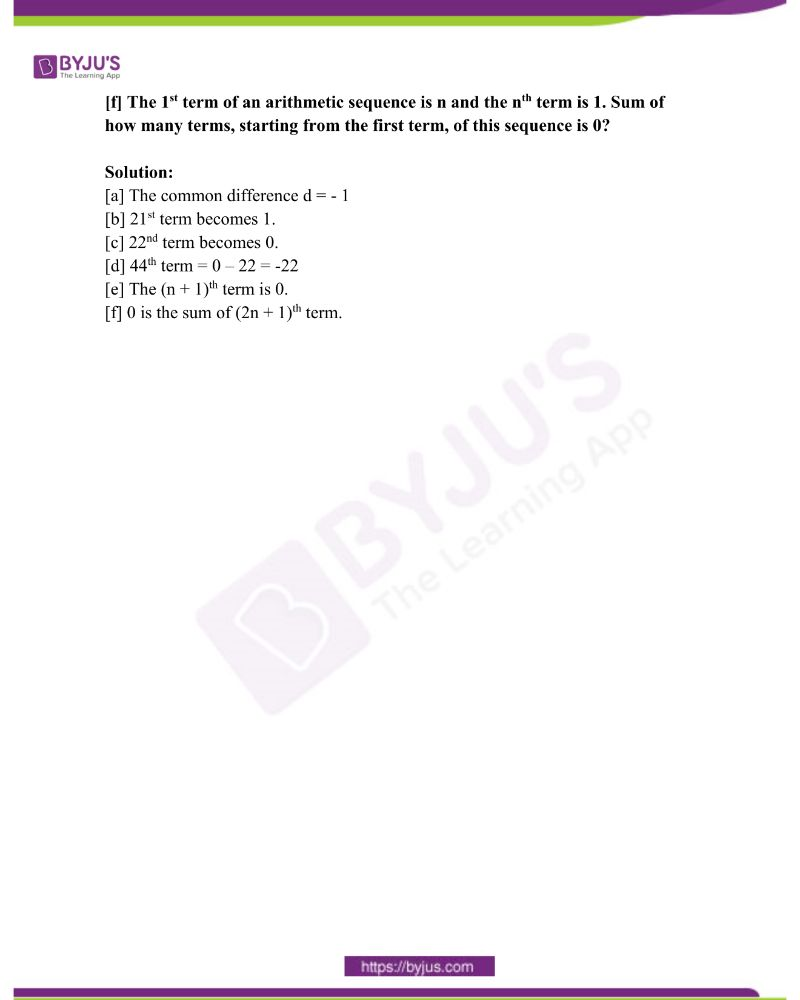Answer any three questions from 1 to 4. Each question carries 2 scores.

[3 * 2 = 6]

Question 1: [a] Write the 6th term of the arithmetic sequence 1, 25, 49, 73, 97 …..

[b] How many perfect square terms are there in the arithmetic sequence 97, 73, 49 …..?

Solution:

[a] Given an arithmetic sequence is 1, 25, 49, 73, 97 , ………….

First term (f) = 1

d = 25 – 1 = 24.

T6 = a + (n – 1)d

6th term = f + 5d

⇒ 1 + 5 × 24

⇒ 1 + 120 = 121

[b] Given an arithmetic sequence is 97, 73, 49, ……….

The perfect square numbers are 1, 4, 9, 16, ……….

Hence the given sequence is 97, 73, 49, 25, 1.

From the above sequence, the perfect square numbers are 49, 25, and 1.

∴ The number of perfect square terms are 3.

Question 2: Chords AB and CD are intersecting at P. AB = 10cm, PB = 4cm, PD = 3cm.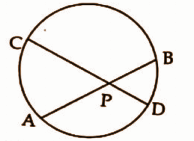[a] What is the length of PA?

[b] Find the length of the PC.

Solution:

Given:

AB = 10cm

PB = 4cm

PD = 3cm

[a] PA = AB – PB

= 10 – 4

= 6cm

[b] PC × PD = PA × PB

PC = [PA × PB] / [PD]

= [6 × 4] / 3

= 8cm

Question 3: Write the polynomial p(x) = x2 – 4 as the product of two first degree polynomials.

Solution:

Given polynomial = p(x) = x2 − 4

First-degree polynomial x2 − 4

= (x + 2) ( x – 2)

Question 4: In the figure, O is the centre of the circle and x2 + y2 = 25 is the equation of the circle.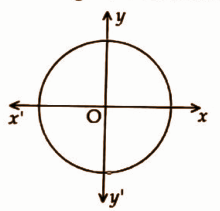[a] What is the radius of the circle?

[b] Write the equation of the circle whose centre is at origin and the radius is 3.

Solution:

Given circle is x2 + y2 = 25

[a] x2 + y2 = r2

r2 = 25

r = √25 = 5

∴ r = 5

The radius of the circle is 5 units.

Hence the equation of the circle = x2 + y2 = r2.

⇒ x2 + y2 = 32

⇒ x2 + y2 = 9

Answer any five questions from 5 to 11. Each question carries 3 scores. [5 * 3 = 15]

Question 5: [a] Write the first term and the common difference of the arithmetic sequence whose algebraic expression is 3n + 5.

[b] The first term of an arithmetic sequence is 8 and the common difference is 5. Write its algebraic form.

Solution:

Given xn = 3n + 5

[a] If n = 1, then the first term can be obtained.

First term = 3 × 1 + 5 = 3 + 8 = 8

Common difference = 3 [∵ coefficient of n be the d ] [b] Given f = 8

d = 5

xn = dn + f – d)

= 5n + (8 – 5)

= 5n + 3

Question 6: In the figure, ∠ABC = 90o, ∠C = ∠D = 45o, AB = 10cm.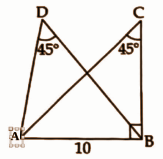[a] What is the length of AC?

[b] What is the radius of the circumcircle of triangle ABC?

[c] What is the radius of the circumcircle of triangle ABD?

Solution:

In right △ ABC, the angles are 450, 450, 900

⇒ 1 : 1 : √2

⇒ AB : BC : AC

⇒ x : x : x √2

⇒ 10 : 10 : 10√2

[a] The length of AC = 10√2 cm.

[b] The radius of the circumcircle of △ABC

= Half of the hypotenuses AC

= 10 √2 / 2

= 5√2 cm

[c] The radius of the circumcircle of △ABD

= Half of the hypotenuses AC

= 10 √2 / 2

= 5√2 cm

Question 7: Draw a circle of radius 3cm. Mark a point P at a distance of 6cm from the centre of the circle. Draw tangents from P to the circle.

Solution: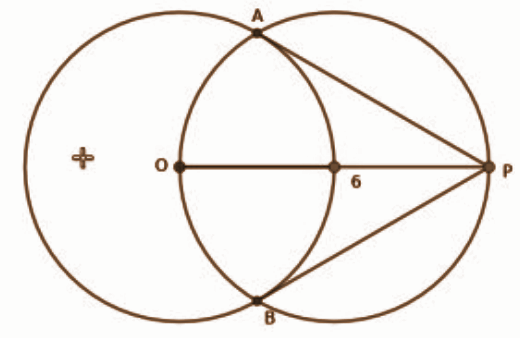Question 8: [a] What is the common difference of the arithmetic sequence x – 1, x, x + 1, …..

[b] If x – 1 is an even number, what is the next even number?

[c] Prove that the product of 2 consecutive even numbers added to 1 gives a perfect square.

Solution:

[a] Given sequence x – 1 , x, x + 1 , ……

d = x – (x – 1)

= x – x + 1

d = 1

[b] Given even number = x – 1

Next even number

= x – 1 + 2

= x + 1

[c] Let consecutive two even number be (x – 1 ) and (x+ 1)

By question, the product of two consecutive numbers is + 1,

(x – 1) (x+ 1) 1 = x1 – 1 + 1 = x2

Here x2 being a perfect square.

Question 9: In the figure, ABCD is a square. Its diagonals are parallel to the coordinate axes. AC = 6 and the coordinates of A is (3, 2). Write the coordinates of the vertices of C, B and D.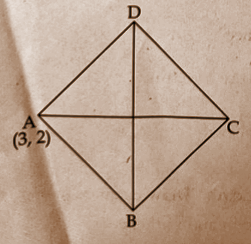Solution:

Given, the coordinates of A = (3, 2)

AC be parallel to the x-axis.

∴ The coordinates of C

= (3 + 6, 2)

= (9, 2)

The coordinates of the midpoint of AC = (6,2)

It is known that the diagonals are equal in a square.

∴ The coordinates of B

= (6, 2 – 3)

= (6, -1)

The coordinates of D

= (6, 2 + 3)

= (6, 5)

Hence the coordinates of A = (3, 2)

The coordinates of B = (6, -1)

The coordinates of C = (9, 2)

The coordinates of D = (6, 5)

Question 10: In the figure, ABCD is a cyclic quadrilateral. Also, ∠A + ∠D = 210o, ∠D + ∠C = 250o.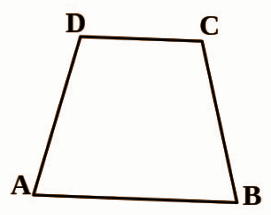[a] What is ∠A + ∠C?

[b] Find the measures of ∠A and ∠C.

Solution:

Given that ∠A + ∠D = 210o, ∠D + ∠C = 250o,

[a] A + C = 1800 (cyclic quadrilateral)

A + D = 210 → (1)

D + C = 250 → (2)

⇒ A + C + 2D = 460

⇒ 180 + 2D = 460

⇒ 2D = 280

D = 1400

[b] A = 210 – 140

A = 700

C = 250 – 140

C = 1100

Question 11: The figure of a square sheet paper is shown below. Length of one side of the paper sheet is 36cm and AB = 10cm. The shaded portion is cut out and folded into a square pyramid.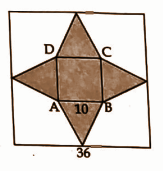[a] What is the length of the base of the pyramid?

[b] What is the slant height of the pyramid?

[c] Find the lateral surface area of the pyramid.

Solution:

Given, Side of the paper sheet = 36cm

AB = 10cm

[a] Base edge of the pyramid

AB = 10cm

[b] Slant height of the pyramid

= [36 − 10] / 2

= 26 / 2

= 13cm [∵ a + 2l = 36, side of the larger square] [c] Lateral surface area = 2al

= 2 × 10 × 13

= 260 cm2

Answer any seven questions form 12 to 21. Each question carries 4 scores. [7 * 4 = 28]

Question 12:

[a] What is the sum of the first 5 terms of the arithmetic sequence 1, 3, 5, 7 …..?

[b] What is the sum of the first n terms of the arithmetic sequence 1, 3, 5, 7 …..?

[c] Find the sum of the first n terms of the arithmetic sequence (1 / n), (3 / n), (5 / n), (7 / n) ……

[d] What is the sum of the first 2020 terms of the arithmetic sequence (1 / 2020), (3 / 2020), (5 / 2020), (7 / 2020)……

Solution:

[a] Given sequence = 1, 3, 5, 7, ……

Sum = n2

Here n = 5

∴ The sum of the first 5 terms

= 52

= 25

[b] The sum of the first n terms = n2

[c] Given sequence = (1 / n), (3 / n), (5 / n), (7 / n) ……

The sum of the first n terms = n2 / n = n

[d] Given sequence (1 / 2020), (3 / 2020), (5 / 2020), (7 / 2020)……

The sum of the first n terms = n2 / n = n

The sum of the first 2020 terms

= n

= 2020

Question 13: Draw a rectangle of length 4cm and breadth 2cm. Draw a square having the same area of the rectangle.

Solution: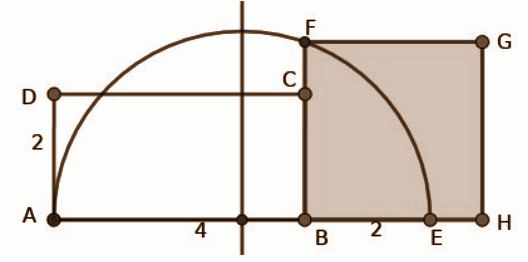• Construct a rectangle ABCD with length 4cm and breadth 2cm.
• Extend the line AB to BE, such that BE = BC.
• Draw a semi-circle with AE as diameter.
• Draw a parallel line BF through B and then BF as length, draw the square BFGH.

Question 14: In a school, the total number of students in 10 A division is equal to the number of students in 10 B. One student is to be selected from each division. The number of boys in 10 A is 20. The probability of selecting a boy from 10 A is (2 / 5) and that of class B is (3 / 5).

[a] How many students are there in 10 A?

[b] What is the probability of selecting a girl from 10 A?

[c] How many boys are there in 10 B?

[d] What is the probability of both the selected students being boys?

Solution:

 Class XA XB Boys 20 30 Girls 30 20 Total 50 50

Given the probability of boys in XA = 2 / 5

Given the probability of boys in XB = 3 / 5

[a] Number of boys in XA

= 20 × [5 / 2]

= 50

[b] Probability of girl from XA

= 1 − [2 / 5]

= [5 − 2] / 5

= 3 / 5

[c] Number of boys in X B

= 50 × [3 / 5]

= 10 × 3

= 30

[d] Both being boys

= [2 / 5] × [3 / 5]

= 6 / 25

Question 15: Perimeter of the rectangle in the figure is 36cm. AC = √164 cm.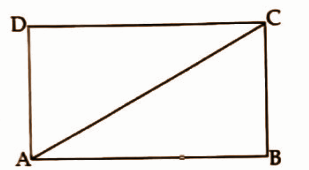[a] What is AB + BC?

[b] Find the length of AB.

Solution:

Given the perimeter = 36cm

AC = √164 cm

[a] 2 (l + b) = 36

∴ AB + BC = 36 / 2

= 18cm

[b] Let AB = x , BC = 18 – x

Since ∆ABCis right-angled, according to Pythagoras theorem,

AC2 = AB2 + BC2

164 = x2 + (18 – x)2

x2+ 324 – 36x + x2 = 164

2x2 – 36x = 164 – 324 = – 160

Dividing by 2

x2 – 18x = – 80 [ square completion method]

x2 – 18x + 81 = – 80 + 81

(x – 9)2 = 1

x – 9 = ± 1

x – 9 = 1 or x – 9 = -1

x = 10 or = 8

So, AB = 10 cm.

Question 16: In triangle ABC, ∠A = ∠B = 30o, AC = 4cm.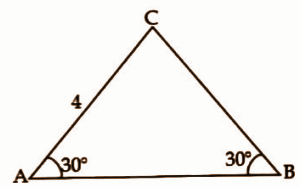[a] What is the length of BC?

[b] Find the length of AB.

[c] In triangle PQR, PQ = 4√3 cm, ∠P = ∠Q = 30o.

Draw the triangle.

Solution: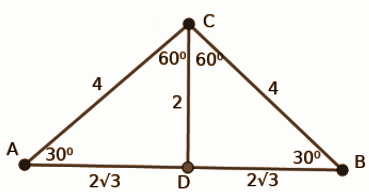Given ,

A = B = 300

AC = 4cm

Draw CD ⊥ AB.

300 : 600 : 900

1 : √3 : 2

x : x√3 : 2x

2 : 2√3 : 4

DC = 2

AC = 4

[a] Length of BC = AC = 4cm

[b] Length of AB = AD + DB

= 2√3 + 2√3

= 4√3cm

[c] Draw PR = 4cm and make R be 1200 and join PQ. △PRQ is the required triangle.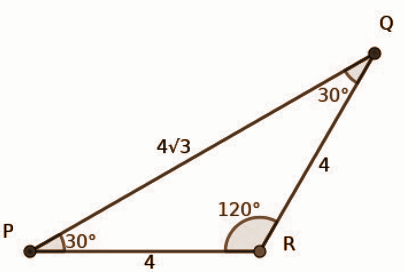Question 17:

[a] If p(x) = x2 – 7x + 13, what is p(3)?

[b] Write the polynomial p(x) – p(3) as the product of two first degree polynomials.

[c] Find the solutions of the equation p(x) – p(3) = 0.

Solution:

[a] Given polynomial

p(x) = x2 – 7x + 13

p(3) = 32 − 7 × 3 + 13

= 9 – 21 + 13

= 1

[b] p(x) – p(3) = x2 – 7x + 13 – 1

= x2 − 7x + 12

= (x – 3) (x – 4)

Hence the product two first degree polynomial = (x – 3) (x – 4)

[c] p(x) – p(3) = 0

x2 – 7x + 12 = 0

⇒ (x – 3) (x – 4) = 0

⇒ (x – 3) = 0 or (x – 4 ) = 0

ie., x = 3 or x = 4.

Hence the solution is x = 3 and 4.

Question 18: In the figure, O is the centre of both circles. AB and AC touch the small circle at P and Q. A, B and C are points on the larger circle.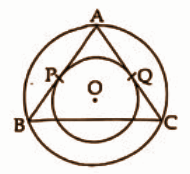[a] If AP = 5cm, then what is the length of AQ?

[b] Prove that AB = AC.

[c] If AP = 5cm and ∠A = 90o, then what is the radius of the small circle?

Solution:

[a] Given AP = 5 cm

Hence the length of AQ = 5cm. [∵ Same tangents from A] [b] AB and AC are tangents

OP ⊥ AB and OQ ⊥ AC [∵ Chord bisector theorem]

AP = BP and AQ = QC

AB = AC

[c] Given A = 900

So it can be seen that APOQ is a square.

[ ∵ OP and OQ be radii ∴ APO = AQO = 900. ie., POQ = 900]

Hence the radius of the small circle = 5cm.

Question 19: Draw the coordinate axes and mark the points A (-3, 0), B (3, 0), C (0, 3√3).

Solution: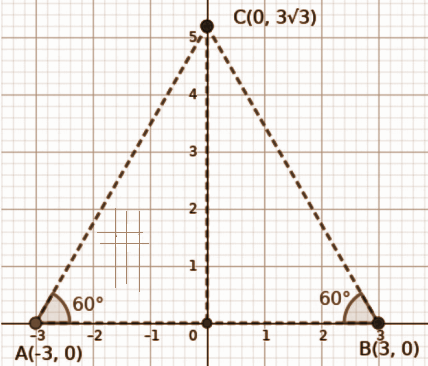Question 20: A sector of radius 12cm and the central angle 120ois rolled up to a cone.

[a] What is the slant height of the cone?

[b] Find the radius and height of the cone.

[c] What is the central angle of the sector to be used to make a cone of base radius √2 cm and height 4cm?

Solution:

[a] The radius of the sector = 12cm [The radius of the sector to be the slant height of the cone ] [b] r / l = x0 / 360o

⇒ r / 12 = 120 / 360

⇒ 360r = 12 × 120

⇒ r = [12 × 120] / 360

∴ r = 4cm

h = √l2 − r2

= √122 −42

= √144 − 16

= √128

= 8√2 cm

[c] r / l = x0 / 360o

Central angle (x0) = [360 × r] / l

To find ‘l’

l = √h2 + r2

r = √2 , h = 4cm

∴ l = √42 + √22

= √16 + 2

= √18

= 3√2

∴ Center angle (x0) = 360×rl

= [360 × √2] / [3√2]

= 1200

Question 21:

[a] What is the slope of the line passing through the points (5, 0) and (3, 2)? Write the equation of the line.

[b] The x coordinate of a point on the line x – y = 5 is 5. What is the y coordinate of that point?

[c] Write the coordinates of the point of intersection of the lines x + y = 5 and x – y = 5.

Solution:

[a] Given points are (5, 0) and (3, 2).

Slope = [y2 − y1] / [x2 − x1]

= [2 − 0] / [3 − 5]

= 2 / −2

= – 1

Equation of the line is

y – y1 = m( x – x1)

= y – 0

= -1 ( x – 5)

y = -x + 5

x + y – 5 = 0 is the equation.

[b] If x = 5, then

5 – y = 5

-y = 5 – 5

= 0

The y coordinate of that point is 0.

[c] Given x + y = 5 and x – y = 0.

x + y = 5 → (1)

x – y = 0 → (2)

Solve (1) and (2),

x and y = 5, and 0

So the coordinates = (5, 0).

Answer any five questions from 22 to 28. Each question carries 5 scores. [5 * 5 = 25]

Question 22: Sum of the first four terms of an arithmetic sequence is 72. Sum of first n terms is also 72.

[a] What is the 5th term of the sequence?

[b] Find the sum of the first five terms.

[c] Write the sequence.

Solution:

Given sum of the first 4 terms = 72

Sum of the first 9 terms = 72

[a] 5th term (x5) = (S9) / 9

= 72 / 9

= 8

[b] Sum of the first 5 terms (S5)

= S4 + x5

= 72 + 8

= 80

[c] X3 = (S5) / 5

= 80 / 5

= 16

X3 + 2d = X5

16 + 2d = 8

2d = – 8

d = – 4

X1 = X3 – 2d

= 16 – [2 × – 4]

= 24

The sequence = 24, 20, 16, 12, 8,…..

Question 23: A boy standing at the edge of a canal sees the top of a tree on the other edge at an elevation of 60o. Stepping 12m back, he sees it at an elevation of 30o. Find the height of the tree.

Solution: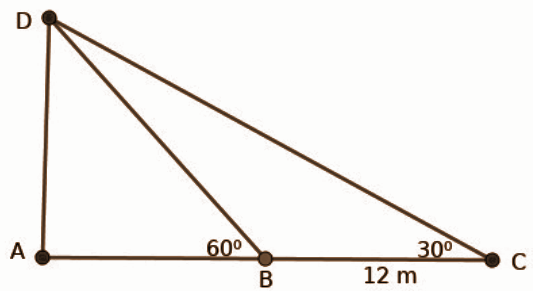Let AB be the height of the tree.

B be the first position of the boy.

C be the second position of the boy.

BC = 12

∠C = 300

∠BPA = 600

∠PBA = 300

∠A = 900

△CBD is an isosceles triangle.

∴ BC = BD = 12

From right △BAP,

300 ; 600 ; 900

1 : √3 : 2

⇒ AD : AB : BD

⇒ x : x√3 : 2x

⇒ 6 : 6√3 : 12

∴ AB = 6√3

Hence the height of the tree = 6√3m.

Question 24: In △ABC, AB = 5cm, ∠A = 650, ∠B = 550. Draw the triangle ABC and the incircle. Measure the radius of the incircle.

Solution: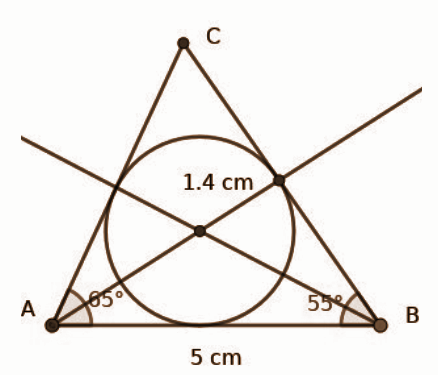Question 25: A circle is drawn with (5, 3) as a centre. (5, 6) is a point on the circle.

[a] What is the radius of the circle?

[b] Write the equation of the circle.

[c] What is the distance from the centre of the circle to the x-axis?

[d] What is the length of the tangents from the origin to the circle?

Solution:

[a] Radius of the circle = 6 – 3 = 3 units

[b] Equation of the circle

= (x – a)2 + (y – b)2 = r2

⇒ (x – 5)2 + (y – 3)2 = 32

⇒ x2 – 10x + 25 + y2 – 6y + 9 = 9

⇒ x2 + y2 – 10x – 6y + 25 = 0

[c] The distance from the centre of the circle to the x-axis is the radius of the circle = 3 units.

[d] The length of the tangents from the origin to the circle = 5 units. [The x-axis being itself a tangent.]

Question 26:

[a] The radius of a solid sphere is 6cm. Find its volume and surface area.

[b] It is cut into two equal halves. What is the total surface area of each hemisphere? What is the volume of a hemisphere?

Solution:

Volume = [4 / 3] πr3

= [4 / 3] π63

= 288π cm3

Total Surface Area = 4πr2

= 4π × 62

= 144πcm2

[b] Total Surface Area of hemisphere = 3πr2

= 3π × 62

= 108πcm2

The volume of hemisphere = [2 / 3] πr3

= [2 / 3] π63

= 144πcm3

Question 27: The table below shows, children of a class sorted according to their marks in the examination.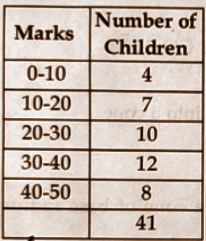[a] If we arrange the children from the one with the least mark to the one with the greatest, then what will be the assumed mark of the 12th student?

[b] Compute the median mark.

Solution:

 Class Frequency Marks Cumulative Frequency 0 – 10 4 <10 4 10 – 20 7 <20 11 20 – 30 10 <30 21 30 – 40 12 <40 33 40 – 50 8 <50 41 Total 41
[a] Assumed mark of the 12th student

= 20 + [30 − 20] / [10 × 2]

= 20 + 1 / 2

= 20.5

[b] N / 2 = 41 / 2 = 20.5

Median class = 20 – 30

l = 20

F = 11

f = 10

Median = l + [(N / 2 − F] / f) / c

= 20 + ([20.5 − 11] / 10) × 10

= 20 + [9.5 / 10] × 10

= 20 + 9.5

= 29.5

∴ Median mark = 29.5

Question 28: In the figure, O is the centre of the large circle. The centre of the small circle is C. OP is a tangent to the small circle. ∠BOQ = 50o.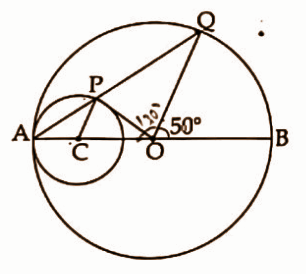[a] ∠OAQ = ?

[b] ∠OCP = ?

[c] ∠APO = ?

[d] ∠POQ = ?

Solution:

Given BOQ = 500

[a] Since △AOQ is isosceles, their base angles are equal.

AOQ = 180 – 50 = 130

A = Q = [180 − 130] / 2

= 50 / 2

= 250

OAQ = 250

[b] OCP = 250 × 2 = 500

[c] APO = 250 + 90o = 1150

[d] POQ = 1800 – ( 50 + AOP)

= 180o – 50o – 40o

= 900

Question 29: Read the following passage. Understand the mathematical concept in it and answer the questions that follow. Each question carries 1 score. [6 * 1 = 6]

The common difference of the arithmetic sequence 15, 14, 13, 12, 11, 10 ….. is 14 – 15 = -1. The first term of the sequence is 15 and the 15th term is 15 + 14 * -1 = 15 – 14 = 1. Similarly, the 4th term is 12 and the 12th term is 4. Its 16th term is x16 = 15 + 15 * -1 = 15 – 15 = 0. So, the sum of the first 31 terms is also 0. That is if the nth term of an arithmetic sequence with common difference -1 is m, then the mth term is n and the (m + n)th term is 0.

[a] 7th term of an arithmetic sequence is 10 and the 10th term is 7. What is the common difference?

[b] What is the 21st term of the arithmetic sequence 21, 20, 19….

[c] 5th term of an arithmetic sequence is 17 and the 17th term is 5. Which term of the sequence is 0?

[d] 5th term of an arithmetic sequence is 17 and the 17th term is 5. What is the 44th term?

[e] 1st term of an arithmetic sequence is n and the nth term is 1. What is the (n + 1)th term?

[f] The 1st term of an arithmetic sequence is n and the nth term is 1. Sum of how many terms, starting from the first term, of this sequence is 0?

Solution:

[a] The common difference d = – 1

[b] 21st term becomes 1.

[c] 22nd term becomes 0.

[d] 44th term = 0 – 22 = -22

[e] The (n + 1)th term is 0.

[f] 0 is the sum of (2n + 1)th term.